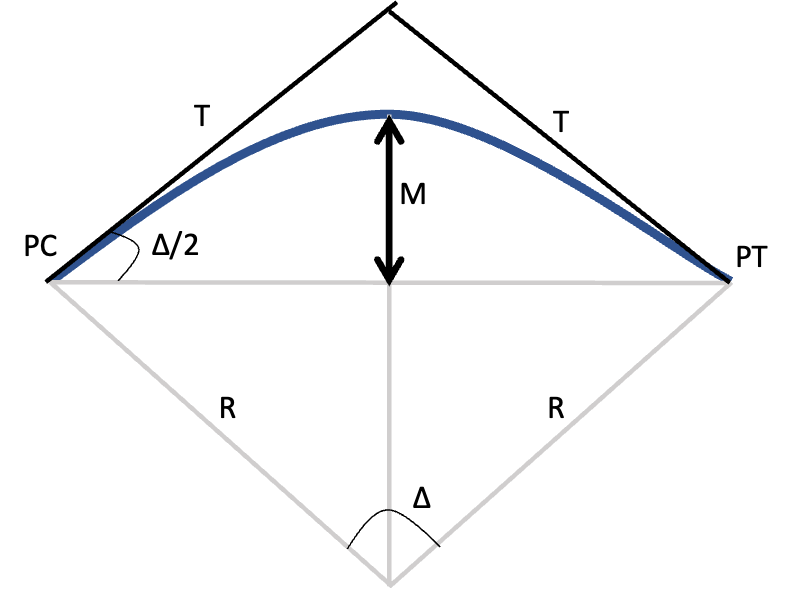# Practice Question: Find the Middle Ordinate on a Horizontal Curve

NCEES Civil PE Specification Geometrics: Basic Circular Curve Elements

Problem

What is most nearly the middle ordinate “M” of the shown standard horizontal cure providing the following curve data:
$$R=1500\ ft$$
$$T=197\ ft$$